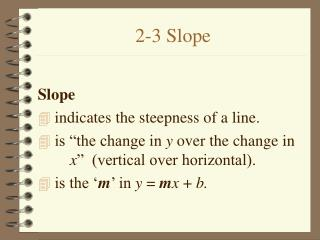DownloadDownload Presentation2-3 Slope

# 2-3 Slope

Télécharger la présentation## 2-3 Slope

- - - - - - - - - - - - - - - - - - - - - - - - - - - E N D - - - - - - - - - - - - - - - - - - - - - - - - - - -
##### Presentation Transcript

1. 2-3 Slope Slope • indicates the steepness of a line. • is “the change in y over the change in x” (vertical over horizontal). • is the ‘m’ in y = mx + b.

2. Finding the Slope of a Line • m = • m = • We use the first equation when given a graph, the second when given two points.

3. x x y y Slope • If a line rises from left to right (uphill), it has a positive slope. • If a line falls from left to right (downhill), it has a negative slope.

4. x x y y Slope • If a line is vertical, it has an undefined slope. (Remember VUX – vertical/undefined/ x = #). • If a line is horizontal it has a slope equal to 0. (Remember HOY – horizontal/zero/y = #).

5. y Find the Slope • To find the slope of this line we will use rise/run. • Pick either point. • We find the rise by counting up (+) or down (-). • Rise is 3 up from the bottom or -3 down from the top point. x

6. x y Find the Slope • To find the run, we count right (+) or left (-). • If you started from the top point, your run will be left -2, if you started at the bottom point, your run will be right 2.

7. x y Find the Slope • So if you started with the top point, your slope would be -3/-2. • If you started with the bottom point, your slope would be 3/2. • Are these the same? Yes!! The slope of the line is 3/2.

8. x x y y Find the Slope • m = • m =

9. x x y y Find the Slope • m= undefined • m = 0

10. Given the points (5, 1) & (6, 4) m = = = 3 Given the points (-6, -2) & (-1, 0) m = Find the Slope of the Line

11. What do you know about the slope of parallel lines? Parallel lines have the SAME SLOPE! What is the slope of the line parallel to y = -2x + 4? m = -2 Parallel Lines

12. What do you know about the slope of perpendicular lines? Perpendicular lines have slopes that are opposite reciprocals! What is the slope of the line perpendicular to y = -2x + 4? m = 1/2 Perpendicular Lines

13. x y Graphing a Line Given a Point & Slope • Graph a line though the point (2, -6) with m=2/3 • Graph (2, -6) • Count up 2 for the rise, and to the right 3 for the run • Plot the point, repeat, then connect

14. Graphing Lines • Graph the line perpendicular to y = 2x + 3 that goes through the point (-2, 3). • The slope of the line is 2 so the slope of the perpendicular line is -1/2. • m = -1/2 b = 3

15. x y Graphing Lines • m = - ½ b = 3 • Plot y-intercept (b) • Use the slope to find two more points • Connect

16. Graphing Lines • Graph the line parallel to x = -1 that goes through the point (3, -3). • The slope of the line is undefined (VUX) so the slope of the parallel line is undefined.

17. (3, -3) m = undefined x y Graph the Line